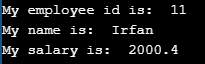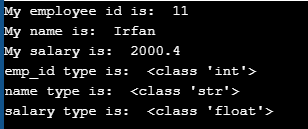# Data Types in Python

## Data Types in Python with Examples

In this article, I am going to discuss Data Types in Python with Examples. Please read our previous article where we discussed Variables in Python with examples. At the end of this article, you will understand the following pointers in detail.

1. What is data type?
2. Different types of data types
3. Built-in data types
4. Sequences in Python
5. Accessing range values
6. User-defined data types
7. Converting from one data type into another data type.
8. Multiple Programs to understand the above concepts
##### What is a Data Type?

All programming languages’ aim is to create some data or to do some actions on the data and process the data. The data can be categorized into different types like integers, float numbers, boolean, string, etc. Categorizing the data is important in order to perform the operations on it. That classification, which states the type of data stored in the variable is called data type. Each and every programming language provides certain data types such that all the types of data can be stored in those.

###### Example: To print different kinds of variables
```emp_id = 11
name = 'Irfan'
salary = 2000.40

print("My employee id is: ", emp_id)
print("My name is: ", name)
print("My salary is: ", salary)```
###### Output:##### type() function in Python:

type() is an in-built or pre-defined function in python that is used to check the data type of the variables. The following example depicts the usage.

###### Example: To print different kinds of variables and their types
```emp_id = 11
name = 'Irfan'
salary = 2000.40

print("My employee id is: ", emp_id)
print("My name is: ", name)
print("My salary is: ", salary)

print("emp_id type is: ", type(emp_id))
print("name type is: ", type(name))
print("salary type is: ", type(salary))```
###### Output:##### Different types of data types in Python with Examples

Generally, data types can be classified into two types:

1. Built-in data types: Data types that are already available in python are called built-in data types
2. User-defined data types: Data types that are created by the programmer
##### Built-in data types in Python:
1. Numeric types (int, Float, Complex)
2. bool
3. None
4. Str
5. Bytes
6. Bytearray
7. Tuple
8. List
9. Range
10. Set
11. dict
##### Numeric types in Python:

int: The int data type represents values or numbers without decimal values. In python, there is no limit for the int data type. It can store very large values conveniently.

###### Example: to print integer value
```emp_id = 11
print(emp_id)
print(type(emp_id))```

Output:
11
<class ‘int’>

Note: In Python 2nd version long data type was existing but in python 3rd version long data type was removed

##### Float Data Type in Python:

The float data type represents a number with decimal values. floating-point numbers can also be written in scientific notation. e and E represent exponentiation. where e and E represent the power of 10. For example, the number 2 * 10pow2 is written as 2E2, such numbers are also treated as floating-point numbers.

###### Example: To print float value
```salary = 50.5
print(salary)
print(type(salary))```

Output:
50.5
<class ‘float’>

###### Example: Print float values
```a = 2e2
b = 2E2
c = 2e3
print(a)
print(b)
print(c)
print(type(a))```

Output:
200.0
200.0
2000.0
<class ‘float’>

##### Complex Data Type in python:

The complex data type represents the numbers that are written in the form of a+bj or a-bj, here a is representing a real part of the number and b is representing an imaginary part of the number. The suffix small j or upper J after b indicates the square root of -1. The part “a” and “b” may contain integers or floats.

###### Example: To print the complex value
```a = 3+5j
b = 2-5.5j
c = 3+10.5j
print(a)
print(b)
print(c)
print()
print(a+b)
print(b+c)
print(c+a)```

Output:
(3+5j)
(2-5.5j)
(3+10.5j)

(5-0.5j)
(5+5j)
(6+15.5j)

If we compare two complex values with <, <=, >, >= operators then we will get TypeError

###### Example: Comparing two complex numbers
```a = 2 + 1j
b = 100 + 10j
print(a>b)```

Output: TypeError: ‘>’ not supported between instances of ‘complex’ and ‘complex’

##### Bool Data Type (boolean data type) in Python:

The bool data type represents boolean values in python. bool data type having only two values are, True and False. Python internally represents, True as 1(one) and False as 0(zero). An empty string (“ ”) represented as False.

###### Example: Printing bool values
```a = True
b = False
print(a)
print(b)
print(a+a)
print(a+b)```

Output:
True
False
2
1

##### None data type in Python:

None data type represents an object that does not contain any value. If any object has no value, then we can assign that object with None type.

###### Example: Printing None data type:
```a = None
print(a)
print(type(a))```

Output:
None
<class ‘NoneType’>

If any function and method are not returning anything then that method can return None data type. We will learn more about this function and method in upcoming articles.

##### Sequences in python:

Sequences in Python are objects that can store a group of values. The below data types are called sequences.

1. Str
2. Bytes
3. Bytearray
4. List
5. Tuple
6. Range
##### str data type in python:

A group of characters enclosed within single quotes or double quotes or triple quotes is called a string. We will discuss more in the string chapter.

###### Example: Printing string data type
```name1 = "nireekshan"
name2 = """nireekshan"""
print(name1)
print(name2)```

Output:
nireekshan
nireekshan

##### Bytes Data Type in Python:

Bytes data type represents a group of numbers just like an array. It can store values that are from 0 to 256. The bytes data type cannot store negative numbers. To create a byte data type. We need to create a list. The created list should be passed as a parameter to the bytes() function. The bytes data type is immutable means we cannot modify or change the bytes object. We can iterate bytes values by using for loop.

###### Example: creating a bytes data type
```x = [10, 20, 100, 40, 15]
y = bytes(x)
print(type(y))```

Output: <class ‘bytes’>

###### Example: Accessing bytes data type elements using index
```x = [10, 20, 30, 40, 15]
y = bytes(x)
print(y)
print(y)
print(y)
print(y)
print(y)```

Output:
10
20
30
40
15

###### Example: Printing the byte data type values using for loop
```x = [10, 20, 100, 40, 15]
y = bytes(x)
for a in y:
print(a)```

Output:
10
20
30
40
15

###### Example: To check Values must be in range 0,256
```x = [10, 20, 300, 40, 15]
y = bytes(x)```

Output: ValueError: bytes must be in range(0, 256)

###### Example: To check Byte data type is immutable
```x = [10, 20, 30, 40, 15]
y = bytes(x)
y = 30```

Output: TypeError: ‘bytes’ object does not support item assignment

##### bytearray data type in python:

The bytearray data type is the same as the bytes data type, but bytearray is mutable means we can modify the content of bytearray data type. To create a bytearray

1. We need to create a list
2. Then pass the list to the function bytearrray().
3. We can iterate bytearray values by using for loop.
###### Example: Creating bytearray data type
```x = [10, 20, 30, 40, 15]
y = bytearray(x)
print(type(y))```

Output: <class ‘bytearray’>

###### Example: Accessing bytearray data type elements using index
```x = [10, 20, 30, 40, 15]
y = bytearray(x)
print(y)
print(y)
print(y)
print(y)
print(y)```

Output:
10
20
30
40
15

###### Example: Printing the byte data type values using for loop
```x = [10, 20, 00, 40, 15]
y = bytearray(x)
for a in y:
print(a)```

Output:
10
20
30
40
15

###### Example: Values must be in the range 0, 256
```x = [10, 20, 300, 40, 15]
y = bytearray(x)```

Output: ValueError: bytes must be in range(0, 256)

###### Example: Bytearray data type is mutable
```x = [10, 20, 30, 40, 15]
y = bytearray(x)
print("Before modifying y value: ", y)
y = 30
print("After modifying y value: ", y)```

Output:
Before modifying y value: 10
After modifying y value: 30

##### List Data Type in Python:

We can create a list data structure by using square brackets []. A list can store different data types. The list is mutable. We will discuss more in the list chapter.

##### Tuple Data Type in Python:

We can create a tuple data structure by using parenthesis (). A tuple can store different data types. A tuple is immutable. We will discuss more in the tuple chapter.

##### Range Data Type in Python:

We can create a range of values by using the range() function. The range data type represents a sequence of numbers. The range data type is immutable means we cannot modify it once it is created. Range data type values can be printed by using for loop.

###### Example: Creating a range of values using a range
```a=range(5)
print(a)
for x in a:
print(x)```

Output:
range(0, 5)
0
1
2
3
4

Note: A list of values can be created using the range.

###### Example: Creating a list
```a=list(range(1, 10))
print(a)```

Output: [1, 2, 3, 4, 5, 6, 7, 8, 9]

###### Example: Accessing values using index
```a=range(1, 10)
print(a)
print(a)```

Output:
1
2

###### Example: Accessing elements out of range
```a=range(1, 10)
print(a)
print(a)```

Output: IndexError: range object index out of range

##### Set Data Type:

We can create a set data structure by using parentheses symbols (). The set can store the same type and different types of elements. We will learn more in the set article.

##### Dictionary Data Type in Python:

We can create dictionary types by using curly braces {}. The dict represents a group of elements in the form of key-value pairs like a map. We will discuss more in the dictionary article

##### User-defined Data Types in Python:

The data type which is created by the programmers is called “user-defined” data types in python. Examples are class, module, array, etc. We will discuss it in our OOPS article.

##### Fundamental Data Types in Python:

In Python, the following data types are considered as Fundamental Data types,

1. Int
2. Float
3. Complex
4. Bool
5. Str
##### Conversion of Data Types in Python:

Based on requirements, developers can convert from one data type into another data type explicitly, this is called type conversion. Python provides inbuilt functions to convert from one type to another type.

1. int() : convert from other type into int type
2. float() : convert from other type into float type
3. complex() : convert from other type into complex type
4. bool() : convert from other type into bool type
5. str() : convert from other type into str type